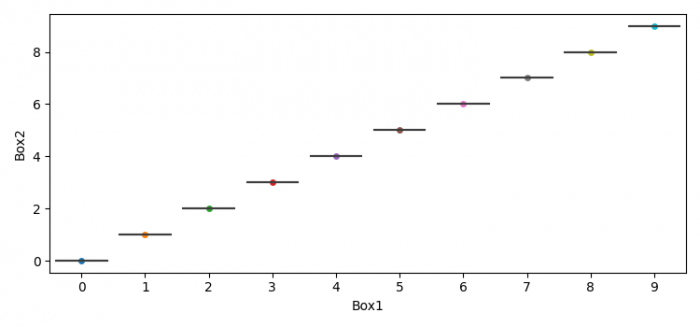# How can box plot be overlaid on top of swarm plot in Seaborn?

MatplotlibPythonData Visualization

To plot a Box plot overlaid on top of a Swarm plot in Seaborn, we can take the following steps −

• Set the figure size and adjust the padding between and around the subplots.
• Create a Pandas dataframe, i.e., two-dimensional, size-mutable, potentially heterogeneous tabular data.
• Initialize the plotter, swarmplot.
• To plot the box plot, use boxplot() method.
• To display the figure, use show() method.

## Example

import seaborn as sns
import matplotlib.pyplot as plt
import pandas as pd
import numpy as np

plt.rcParams["figure.figsize"] = [7.50, 3.50]
plt.rcParams["figure.autolayout"] = True

data = pd.DataFrame({"Box1": np.arange(10), "Box2": np.arange(10)})
ax = sns.swarmplot(x="Box1", y="Box2", data=data, zorder=0)

sns.boxplot(x="Box1", y="Box2", data=data, showcaps=False, boxprops={'facecolor': 'None'}, showfliers=False, whiskerprops={'linewidth': 0}, ax=ax)

plt.show()

## Output